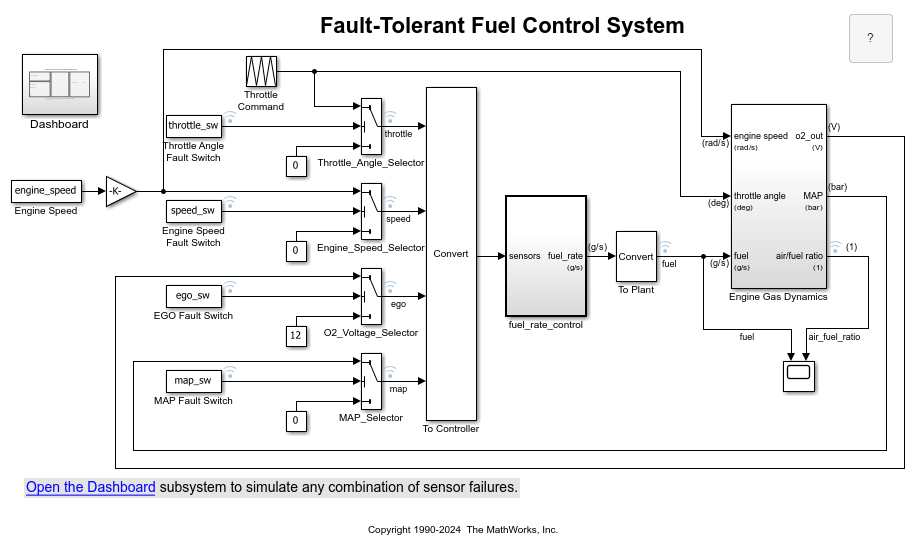# LUTCompressionResult

Optimized lookup table data for all Lookup Table blocks in a system

## Description

A `LUTCompressionResult` object contains the optimized lookup table data for all Lookup Table blocks in a system. To create a `LUTCompressionResult` object, use the `FunctionApproximation.compressLookupTables` function. To replace the lookup tables in your system with the optimized version, use the `replace` function.

## Creation

### Syntax

``CompressionResult = compressLookupTables(system)``
``CompressionResult = compressLookupTables(system, Name,Value)``

### Description

example

````CompressionResult = compressLookupTables(system)` compresses all n-D Lookup Table blocks in the specified system. The compressed Lookup Table blocks output the same numerical results as the original Lookup Table blocks within the bounds of the breakpoints.You can achieve additional memory savings by compressing each Lookup Table block in the model individually and specifying tolerances for the compressed lookup table.```
````CompressionResult = compressLookupTables(system, Name,Value)` compresses all n-D Lookup Table blocks in the specified system with additional properties specified by name and value pair arguments.```

### Input Arguments

expand all

Name of model or subsystem in which to compress all n-D Lookup Table blocks, specified as a character vector.

Example: ```compressionResult = FunctionApproximation.compressLookupTables('sldemo_fuelsys');```

Data Types: `char`

Name-Value Pair Arguments

Specify optional comma-separated pairs of `Name,Value` arguments. `Name` is the argument name and `Value` is the corresponding value. `Name` must appear inside quotes. You can specify several name and value pair arguments in any order as `Name1,Value1,...,NameN,ValueN`.

Whether to display details of each iteration of the optimization, specified as a logical. A value of `1` results in information in the command window at each iteration of the approximation process. A value of `0` does not display information until the approximation is complete.

Data Types: `logical`

Specify the word lengths, in bits, that can be used in the lookup table approximate based on your intended hardware. For example, if you intend to target an embedded processor, you can restrict the data types in your lookup table to native types, 8, 16, and 32. The word lengths must be between 1 and 128.

Data Types: `single` | `double` | `int8` | `int16` | `int32` | `int64` | `uint8` | `uint16` | `uint32` | `uint64` | `fi`

`Simulink.FindOptions` object specifying options for finding lookup tables in the system.

## Properties

expand all

Units for `MaxMemoryUsage` property, specified as `'bits'`, `'bytes'`, or one of the other enumerated options.

Data Types: `char`

Table summarizing the effects of compression. The table contains one row for each lookup table compressed in the system and its corresponding memory savings.

Data Types: `table`

Number of lookup tables found in the specified system, specified as an integer-valued scalar.

Data Types: `double`

Number of lookup tables compressed in the system, specified as an integer-valued scalar.

Data Types: `double`

Total memory of all lookup tables in the system before compression, returned as a scalar. You can specify the units of this property by using the `MemoryUnits` property.

Data Types: `double`

Total memory of all lookup tables in the system after compression, returned as a scalar. You can specify the units of this property by using the `MemoryUnits` property.

Data Types: `double`

Difference between the total memory of all lookup tables in the system before and after compression, returned as a scalar. You can specify the units of this property by using the `MemoryUnits` property.

Data Types: `double`

Percentage reduction in the memory used by the lookup tables in the system after compression, returned as a scalar.

Data Types: `double`

System containing compressed lookup tables, returned as a character vector. `SUD` is the same as the `system` input argument of the `FunctionApproximation.compressLookupTables` function.

Data Types: `char`

Word lengths used for breakpoints and table data in the compressed lookup tables, returned as a scalar or vector of integers.

Data Types: `double`

`Simulink.FindOptions` object specifying options for finding lookup tables in the system.

## Object Functions

 `replace` Replace all Lookup Table blocks with compressed lookup tables `revert` Revert compressed Lookup Table blocks to original versions

## Examples

collapse all

This example shows how to compress all Lookup Table blocks in a system.

Open the model containing the lookup tables that you want to compress.

```system = 'sldemo_fuelsys'; open_system(system) ```Use the `FunctionApproximation.compressLookupTables` function to compress all of the lookup tables in the model. The output specifies all blocks that are modified and the memory savings for each.

```compressionResult = FunctionApproximation.compressLookupTables(system) ```
```- Found 5 supported lookup tables - Percent reduction in memory for compressed solution - 2.37% for sldemo_fuelsys/fuel_rate_control/airflow_calc/Pumping Constant - 2.37% for sldemo_fuelsys/fuel_rate_control/control_logic/Throttle.throttle_estimate/Throttle Estimation - 3.55% for sldemo_fuelsys/fuel_rate_control/control_logic/Speed.speed_estimate/Speed Estimation - 6.38% for sldemo_fuelsys/fuel_rate_control/control_logic/Pressure.map_estimate/Pressure Estimation - 9.38% for sldemo_fuelsys/fuel_rate_control/airflow_calc/Ramp Rate Ki compressionResult = LUTCompressionResult with properties: MemoryUnits: bytes MemoryUsageTable: [5x5 table] NumLUTsFound: 5 NumImprovements: 5 TotalMemoryUsed: 6024 TotalMemoryUsedNew: 5796 TotalMemorySavings: 228 TotalMemorySavingsPercent: 3.7849 SUD: 'sldemo_fuelsys' WordLengths: [8 16 32] FindOptions: [1x1 Simulink.internal.FindOptions] Display: 1 ```

Use the `replace` function to replace each Lookup Table block with a block containing the original and compressed version of the lookup table.

```replace(compressionResult); ```

You can revert the lookup tables back to their original state using the `revert` function.

```revert(compressionResult); ```

### Functions

Introduced in R2020a

## SupportGet trial now